In my study of data structures, I've made it to hash tables. I'm spending a little extra time on this one, because there is some math and inherent gotchas in hashing and I want to fully understand. I may not get all the math, but I've got the important bits.

In addition, hash tables are one of the most used data structures in my career, I was really interested in learning everything I could. It's amazing that I've used these for 15 years and never knew how they were implemented.

There are a couple of hashing functions that are pretty common, and they are in the Universal family of hashing functions.

## Hashing Integers

For integers, the quick and dirty (yet extremely effective) looks like this:In this hashing function, where a and b are randomly chosen integers modulo p with a != 0. P is a prime greater than m, the number of slots your hash table will support.

This was devised by Carter and Wegman in 1977.

## Hashing Strings

Hashing strings is similar to hashing an integer:In this implementation, p again is a prime greater than m, and a is a non-random number, preferable relatively prime. Always avoid powers of 2 in m and a.

Here's my polyhash function:

``````def polyhash_prime(word, a, p, m):
hash = 0
for c in word:
hash = (hash * a + ord(c)) % p

return abs(hash % m)
``````

Above algorithm is also known as Multiplicative hash function. In practice, the mod operator and the parameter p can be avoided altogether by simply allowing integer to overflow because it is equivalent to mod (Max-Int-Value + 1) in many programming languages. Below table shows values chosen to initialize h and a for some of the popular implementations.

Implementation INITIAL_VALUE a
Bernstein's hash function 5381 33
STLPort 4.6.2 0 5
Kernighan and Ritchie's hash function 0 31
`java.lang.String.hashCode()` 0 31

This is very close to what is used in Java's built-in string hashing function. The only difference is that Java doesn't modulo with p, and hashes the string in reverse order.

In my implementation, I chose a = 31, the same as what Java uses in the table above.

## Putting PolyHash to the test

The string hashing function is also known as polyhash, due to polynomial hashing.

I was very curious as to see what kind of distribution occurs when using such as simple hashing algorithm so I did an experiment.

I wrote a Python script that takes each word from Dickens' A Tale of Two Cities, hashes it into a hash range of size N/2, where N is the number of unique words, and tells me the size distribution of hash slots (buckets). Why N/2? So the load factor will be 0.5.

### Random insertion

Since a good hashing function hashes a given item into a hash range with probability 1/m for any slot, I started with a random hashing function that simply returns a random integer between 0 and m-1. That distribution looks like this, with ~10,000 slots: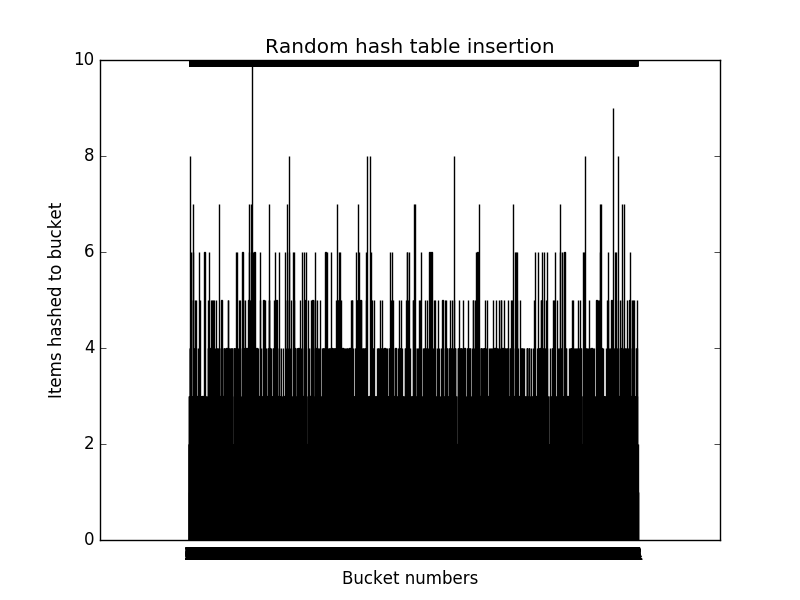That's what we would expect. Here's a more informative bar chart showing sizes of buckets, and how many buckets there are of each size: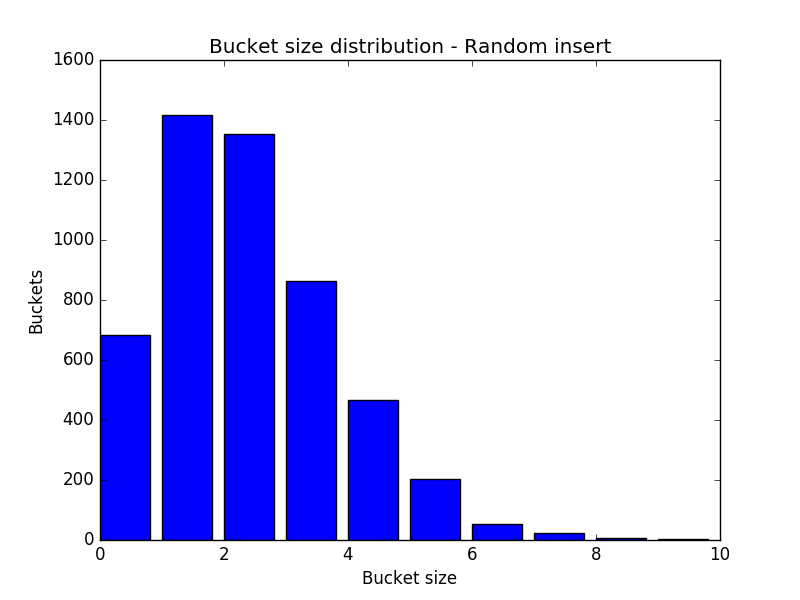So the goal is to have the outcome of an ideal hash function to approximate this distribution. About 7% of the slots will be empty, and most buckets will be size 1-4.

### Polyhash with Modulo Prime

I started with the polyhash as stated above, with a prime and a decent a. I chose 31. It gave this distribution: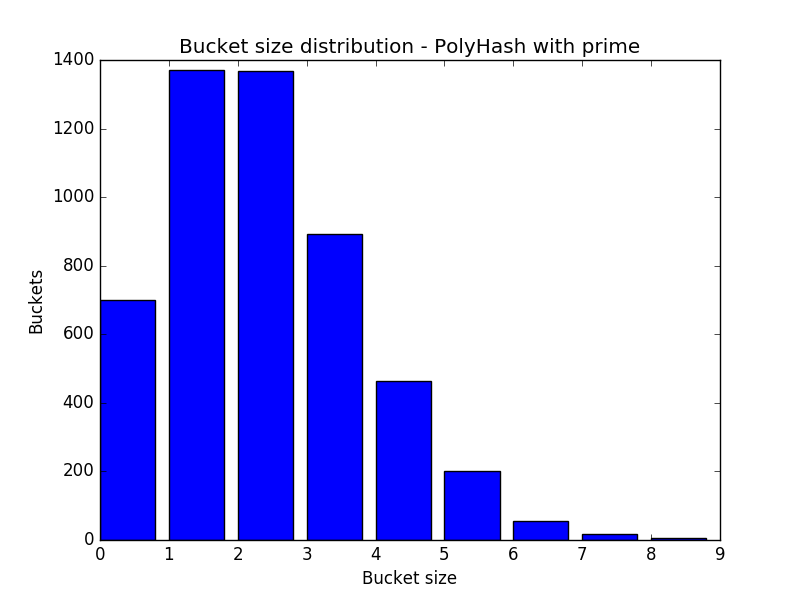As you can see, this distribution is ridiculously close to the random insertion. Amazing. I'm sold. And it's even better, because the max bucket size drops from 11 to 8. Ignore the last number in the graphs.

But what happens when you take out the prime number portion?

### Polyhash without Prime

You get a very similar result, so it seems ignoring a prime has no effect: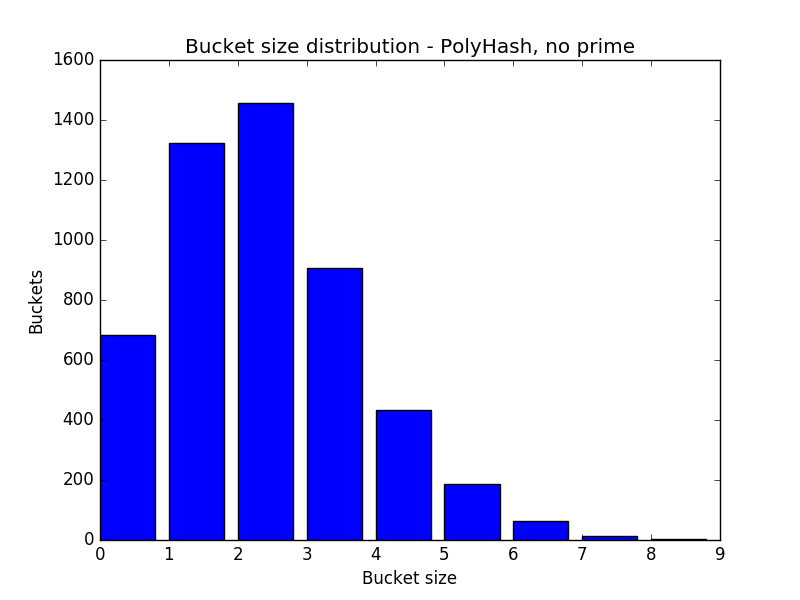Since p is related to m, where p > m, calculating a new prime each time you double or quarter a hash table might be somewhat expensive, and more so as m grows. It's safe to say you can ignore p.

### Messing with the a Parameter

What about that a = 31? What difference does it make? It makes a big difference at low numbers, but then as it grows, the difference quickly disappears. With a=1, 40% of the buckets are empty, and all remaining buckets end up with long chains of keys (large buckets):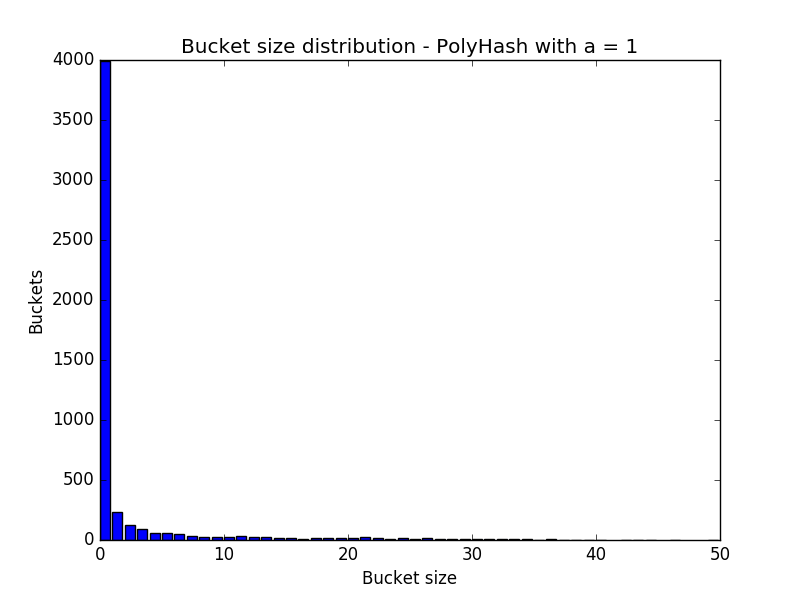Increasing to 2 or 3 quickly evens out the distribution. 2 and 3 are both prime, and 3 looks to be good enough, as it approximates that of polyhash with a=31, but it's such a small number it's not a safe modulo for the domain of characters. 31 is also prime, and it gets you closest to random distribution, and any prime higher doesn't make any improvement.

So 31 it is!

Head over here to see the code:
https://github.com/jwasham/practice-python/blob/master/hashes/hash-test.py